# KSEEB Solutions for Class 9 Maths Chapter 5 Triangles Ex 5.2

KSEEB Solutions for Class 9 Maths Chapter 5 Triangles Ex 5.2 are part of KSEEB Solutions for Class 9 Maths. Here we have given Karnataka Board Class 9 Maths Chapter 5 Triangles Exercise 5.2.

## Karnataka Board Class 9 Maths Chapter 5 Triangles Ex 5.2

Question 1.
In an isosceles triangle ABC, with AB = AC, the bisectors of ∠B and ∠C intersect each other at O. Join A to O. Show that:
(i) OB = OC
(ii) AO bisects ∠A.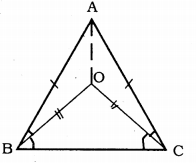Solution:
Data: In an isosceles triangle ABC, with AB = AC, the bisectors of ∠B and ∠C intersect each other at O. Join A to O.
To Prove:
(i) OB = OC
(ii) AO bisects ∠A.
Proof:
(i) In ∆ABC, AB = AC
∴ ∠ABC = ∠ACB
$$\frac{1}{2}$$ ABC = $$\frac{1}{2}$$ ACB
∠OBC = ∠OCB.
In ∆OBC Now, ∠OBC = ∠OCB is proved.
∴ ∆OBC is an isosceles triangle.
∴ OB = OC.

(ii) In ∆AOB and ∆AOC,
AB = AC (Data)
OB = OC (proved)
AO is common.
Side, Side, Side postulate.
∴ ∆AOB ≅ ∆AOC
∴ ∠OAB = ∠OAC
∴ AO bisects ∠A.

Question 2.
In ∆ABC, AD is the perpendicular bisector of BC. Show that ∆ABC is an isosceles triangle in which AB = AC.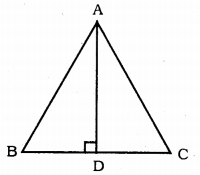Solution: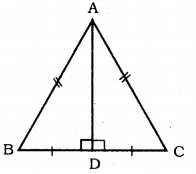Data: In ∆ABC, AD is the perpendicular bisector of BC.
To Prove: ∆ABC is an isosceles triangle in which AB = AC.
Proof: In ∆ABC, AD is the perpendicular bisector of BC.
∴ BD = DC
BD = DC (AD is the perpendicular bisector)
∴ Angles opposite to equal sides of an isosceles triangle are equal.
∴ AB = AC
∴ In ∆ABC, If AB = AC, then
∆ABC is an isosceles triangle.

Question 3.
ABC is an isosceles triangle in which altitudes BE and CF are drawn to equal sides AC and AB respectively. Show that these altitudes are equal.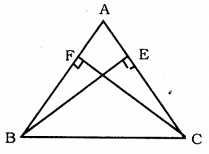Solution:
Data: ABC is an isosceles triangle in which altitudes BE and CF are drawn to equal sides AC and AB respectively
To Prove: Altitude BE = Altitude CF.
Proof: In ∆ABC,
AB = AC and CF ⊥ AB, BE ⊥ AC.
∴ ∠BEC = ∠CFB = 90° (Data)
Angles opposite to equal sides of an isosceles triangle are equal.
BC is common.
∴ ∆BEC ≅ ∆CFB (ASA postulate)
∴ BE = CF.

Question 4.
ABC is a triangle in which altitudes BE and CF to sides AC and AB are equal. Show that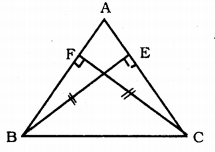(i) ∆ABE ≅ ∆ACF
(ii) AB = AC i.e., ∆ABC is an isosceles triangle.
Solution:
Data: In ∆ABC, altitudes BE and CF to sides AC and AB are equal and BE = CF.
To Prove:
(i) ∆ABE ≅ ∆ACF
(ii) AB = AC i.e., ∆ABC is an isosceles triangle.
Proof: In ∆ABE and ∆ACF,
∠AEB = ∠AFC = 90° (Data)
Altitude BE = Altitude CF (Data)
∠A is Common.
∴ ∆ ABE ≅ ∆ACF (AAS Postulate)
∴ AB = AC
∴ ∆ABC is an isosceles triangle.

Question 5.
ABC and DBC are two isosceles triangles on the same base BC. Show that ∠ABD = ∠ACD.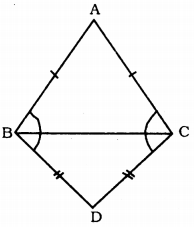Solution:
Data: ABC and DBC are two isosceles triangles on the same base BC.
To Prove: ∠ABD = ∠ACD
Proof: In ∆ABC, AB = AC,
∴ Opposite angle ∠ABC = ∠ACB …………. (i)
Similarly in ∆BDC, BD = DC.
Opposite angle ∠DBC = ∠DCB ………….. (ii)
From (i) and (ii),
∠ABC = ∠ACB
Adding ∠DBC and ∠DCB on both sides,
∠ABC + ∠DBC = ∠ABD
∠ACB + ∠DCB = ∠ACD
Equals are added to equal angles.
∴ ∠ABD = ∠ACD.

Question 6.
∆ABC is an isosceles triangle in which AB = AC. Side BA is produced to D such that AD = AB. Show that ∠BCD is a right angle.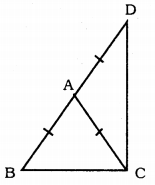Solution:
Data: ∆ABC is an isosceles triangle. AB = AC. Side BA is produced to D such that AD = AB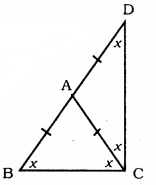To Prove: ∠BCD is a right angle.
Proof: In ∆ABC, AB = AC.
∴ ∠ABC = ∠ACB = x°
Similarly, in ∆ACD,
AB = AC.
Angles opposite to equal sides of a triangle are equal.
∴ ∠ACD = ∠ADC = x.
Now in ∆DCB,
∠B + ∠C + ∠D = 180°
∠DBC + ∠ACB + ∠ACD + ∠ADC = 180°
x + x + x + x = 180
4x = 180
∴ x = $$\frac{180}{4}$$
∴ x = 45°
Now, ∠DCB = ∠DCA + ∠ACB
= x + x
= 2x
= 2 × 45 (∵ x = 45°)
∴∠DCB = 90°
∴ ∠BCD is an right angle.

Question 7.
ABC is a right-angled triangle in which ∠A = 90° and AB = AC. Find ∠B and ∠C.
Solution:
Data: ABC is a right-angled triangle in which ∠A = 90° and AB = AC.
To Prove: ∠B = ? and ∠C = ?
Proof: In ∆ABC, AB = AC, then
∴ ∠B = ∠C.
In ∆ABC, ∠A + ∠B + ∠C = 180°
90 + ∠B + ∠C = 180°
∠B + ∠C = 180 – 90°
∠B + ∠C = 90°
∠B + ∠C = 90°.
But, ∠B = ∠C,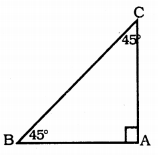∠B + ∠C = 90°
∠B + ∠C = 90°
2∠B = 90°
∴ ∠B = $$\frac{90}{2}$$ = 45
∴ ∠C = 45° ∵∠ABC = ∠ACB

Question 8.
Show that the angles of an equilateral triangle are 60° each.
Solution:
In ∆ABC, AB = AC = BC.
∴ ∠A = ∠B = ∠C, let this is x°.
But sum of three angles is 180°.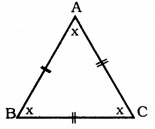∠A + ∠B + ∠C = 180°
x + x + x = 180°
3x = 180°
∴ x = $$\frac{180}{3}$$ = 60°
∴ ∠A = 60°
∠B = 60°
∠C = 60°

We hope the KSEEB Solutions for Class 9 Maths Chapter 5 Triangles Ex 5.2 help you. If you have any query regarding Karnataka Board Class 9 Maths Chapter 5 Triangles Exercise 5.2, drop a comment below and we will get back to you at the earliest.